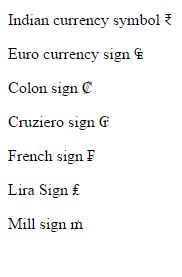# Symbols in HTML5

### Arrow symbols

To add the different technical, mathematical symbols, we can use the HTML to create the symbols

``` <p>Left Arrow &#8592;</p>
<p>Upward Arrow &#8593;</p>
<p>Right Arrow &#8594;</p>
<p>Downward Arrow &#8595;</p>
<p>Leftright Arrow &#8596;</p>
<p>UpDwown Arrow &#8597;</p>
<p>Northwest Arrow &#8598;</p>
<p>NorthEast Arrow&#8599;</p>
<p>South west Arrow&#8601;</p>
<p>Left wave Arrow &#8604;</p>
<p>Right wave Arrow &#8605;</p>
<p>Downwards Arrow with tip leftwards &#8629;</p>
<p>Clockwise semicircle arrow &#8631;</p>
<p>Leftwards paired Arow &#8647;</p>
<p>Leftright double Arrow With stroke &#8654;</p>
<p>Leftwards Double Arrow &#8656;</p>
<p>Rightwards triple Arow &#8667;</p>
<p>Upwards right Arrow &#8679;</p>
<p>Leftrightopened Arrow &#8703;</p>
```

In the above code snippet we have defined the symbols of arrows using html, we are having the code as &#8592 and if we go on increase the numbers we get different styles of the arrows

output### Mathematical symbols

It is used to define the mathmatical symbols

``` <p>For All &#8704;</p>
<p>Complement &#8705;</p>
<p>Plus Minus &#8723;</p>
<p>Division slash;</p>
<p>Bullet opearator &#8729;</p>
<p>Parallel To &#8741;</p>
<p>Angle &#8736;</p>
<p>Logical And &#8743;</p>
<p>Logical OR &#8744;</p>
<p>Union &#8746;</p>
<p>Double integral &#8748;</p>
```

Mathematical symbols

In the above code snippet we have defined the mathematical symbols , we have used the &# numbers to define the mathematical symbols

output### Currency symbols

It is used to define the symbols of the currency of different states

```<p>Indian currency symbol &#8377</p>
<p>Euro currency sign &#8352</p>
<p> Colon sign &#8353</p>
<p>Cruziero sign &#8354</p>
<p>French sign &#8355</p>
<p>Lira Sign &#8356</p>
<p>Mill sign &#8357</p>
```

Currency symbols

In the above code snippet we have defined the curremcy symbols of the different states using &#8377 and differnet numbers to display the signs of the different states currency

outputViews: 3854 | Post Order: 26